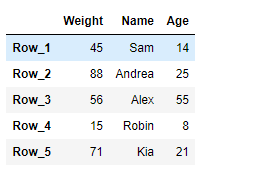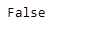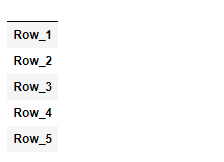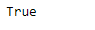# Python | Pandas DataFrame.empty

Pandas DataFrame is a two-dimensional size-mutable, potentially heterogeneous tabular data structure with labeled axes (rows and columns). Arithmetic operations align on both row and column labels. It can be thought of as a dict-like container for Series objects. This is the primary data structure of the Pandas.

Pandas` DataFrame.empty` attribute checks if the dataframe is empty or not. It return `True` if the dataframe is empty else it return `False`.

Syntax: DataFrame.empty

Parameter : None

Returns : bool

Example #1: Use `DataFrame.empty` attribute to check if the given dataframe is empty or not.

 `# importing pandas as pd ` `import` `pandas as pd ` ` `  `# Creating the DataFrame ` `df ``=` `pd.DataFrame({``'Weight'``:[``45``, ``88``, ``56``, ``15``, ``71``], ` `                   ``'Name'``:[``'Sam'``, ``'Andrea'``, ``'Alex'``, ``'Robin'``, ``'Kia'``], ` `                   ``'Age'``:[``14``, ``25``, ``55``, ``8``, ``21``]}) ` ` `  `# Create the index ` `index_ ``=` `[``'Row_1'``, ``'Row_2'``, ``'Row_3'``, ``'Row_4'``, ``'Row_5'``] ` ` `  `# Set the index ` `df.index ``=` `index_ ` ` `  `# Print the DataFrame ` `print``(df) `

Output :Now we will use `DataFrame.empty` attribute to check if the given dataframe is empty or not.

 `# check if there is any element ` `# in the given dataframe or not ` `result ``=` `df.empty ` ` `  `# Print the result ` `print``(result) `

Output :As we can see in the output, the `DataFrame.empty` attribute has returned `False` indicating that the given dataframe is not empty.

Example #2: Use `DataFrame.empty` attribute to check if the given dataframe is empty or not.

 `# importing pandas as pd ` `import` `pandas as pd ` ` `  `# Creating an empty DataFrame ` `df ``=` `pd.DataFrame(index ``=` `[``'Row_1'``, ``'Row_2'``, ``'Row_3'``, ``'Row_4'``, ``'Row_5'``]) ` ` `  `# Print the DataFrame ` `print``(df) `

Output :Now we will use `DataFrame.empty` attribute to check if the given dataframe is empty or not.

 `# check if there is any element ` `# in the given dataframe or not ` `result ``=` `df.empty ` ` `  `# Print the result ` `print``(result) `

Output :As we can see in the output, the `DataFrame.empty` attribute has returned `True` indicating that the given dataframe is empty.

My Personal Notes arrow_drop_upCheck out this Author's contributed articles.

If you like GeeksforGeeks and would like to contribute, you can also write an article using contribute.geeksforgeeks.org or mail your article to contribute@geeksforgeeks.org. See your article appearing on the GeeksforGeeks main page and help other Geeks.

Please Improve this article if you find anything incorrect by clicking on the "Improve Article" button below.

Article Tags :

Be the First to upvote.

Please write to us at contribute@geeksforgeeks.org to report any issue with the above content.